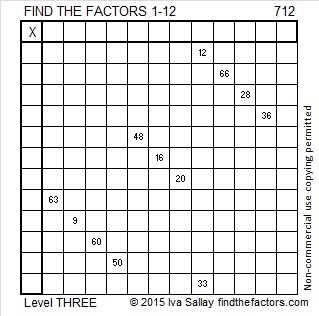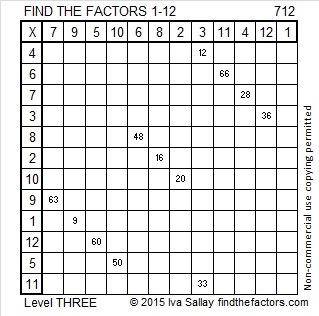# 712 and Level 3

• 712 is a composite number.
• Prime factorization: 712 = 2 x 2 x 2 x 89, which can be written 712 = (2^3) x 89
• The exponents in the prime factorization are 3 and 1. Adding one to each and multiplying we get (3 + 1)(1 + 1) = 4 x 2 = 8. Therefore 712 has exactly 8 factors.
• Factors of 712: 1, 2, 4, 8, 89, 178, 356, 712
• Factor pairs: 712 = 1 x 712, 2 x 356, 4 x 178, or 8 x 89
• Taking the factor pair with the largest square number factor, we get √712 = (√4)(√178) = 2√178 ≈ 26.683328.Here’s a Level 3 puzzle for you to solve:Print the puzzles or type the solution on this excel file: 12 Factors 2015-12-14

——————————————————————————

Here are a few more facts about the number 712:

37 + 38 + 39 + 40 + 41 + 42 + 43 + 44 + 45 + 46 + 47 + 48 + 49 + 50 + 51 + 52 = 712; that’s 16 consecutive numbers.

712 is the sum of the twenty-one prime numbers from 2 to 73.

It is also the sum of consecutive prime numbers 353 and 359.

Because 89 is one of its prime factors, 712 is the hypotenuse of the Pythagorean triple 312-640-712. What is the greatest common factor of those three numbers?

712 is palindrome 4B4 in BASE 12 because 4(144) + 11(12) + 4(1) = 712.

——————————————————————————

A Logical Approach to solve a FIND THE FACTORS puzzle: Find the column or row with two clues and find their common factor. (None of the factors are greater than 12.)  Write the corresponding factors in the factor column (1st column) and factor row (top row).  Because this is a level three puzzle, you have now written a factor at the top of the factor column. Continue to work from the top of the factor column to the bottom, finding factors and filling in the factor column and the factor row one cell at a time as you go.This site uses Akismet to reduce spam. Learn how your comment data is processed.